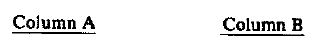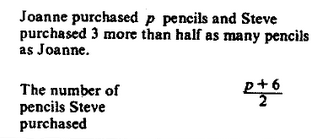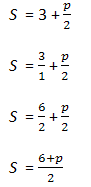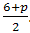# Quantitiative Comparison Word Problem

The Quantitative Comparison question type on the GRE can be very challenging. Essentially, you are given some information (usually in the form of a sentence, equation, or picture) and then two quantities. You are then asked to determine:

A if the quantity in Column A is greater;

B if the quantity in Column B is greater;

C if the two quantities are equal;

D is the relationship cannot be determined from the information given.

Like most questions on the GRE Quantitative section, the concepts involved in solving this question type are not of themselves very advanced, you just have to be careful in their application. Let’s go through an example problem:Word problems require you to translate words into math. You will want to learn key phrases that often come up and their math equivalents. With this problem, we will rewrite the phrase “Steve purchased 3 more than half as many pencils as Joanne” into math. To start, Let the variable S mean the number of pencils Steve purchased.

“Steve purchased 3 more than half as many pencils as Joanne”

S = “3 more than half as many pencils as Joanne”

The phrase “more than” means addition.  The value of 3 is added to “half as many pencils as Joanne.”

S = “3 more than half as many pencils as Joanne”

S  = 3 + “half as many pencils as Joanne”

“Half as many” means half, or divided by two. Remember that the number of pencils purchased by Joanne is represented by p

S  = 3 + “half as many pencils as Joanne”

S  = 3 + “half of p

S  = 3 + (p/2)

Now, simplify by combining 3 and (p/2). Remember that to add fractions, you need a common denominator.The number of pencils Steve purchased, or S, is the same as.  Therefore, the two values in the columns are equal and the answer is C.

Test Masters offers the most comprehensive and successful GRE course available; every Test Masters GRE course, whether it is online or in-class, comes with a 10 point Score Increase Guarantee.

This entry was posted in Example Problems and tagged , , , . Bookmark the permalink.